# Capacitor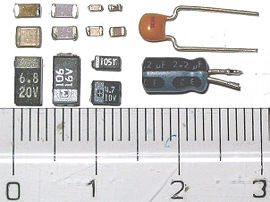Capacitors: SMD ceramic at top left; SMD tantalum at bottom left; through-hole tantalum at top right; through-hole electrolytic at bottom right. Major scale divisions are in centimeters.

A capacitor (or condenser) is an electrical device that can store energy in the electric field between a pair of closely-spaced conductors (called 'plates'). When voltage is applied to the capacitor, electric charges of equal magnitude but opposite polarity build up on each plate. They are used in electrical circuits as energy-storage devices. They can also be used to differentiate between high-frequency and low-frequency signals and this makes them useful in electronic filters.

Capacitors have allowed for the development of many important items in modern life, such as computer circuitry, TVs, and even flash units for cameras.

## History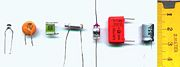Various types of capacitors. From left: multilayer ceramic, ceramic disc, multilayer polyester film, tubular ceramic, polystyrene (twice: axial and radial), electrolytic. Major scale divisions are in centimeters.

In October 1745, Ewald Georg von Kleist (1700-1748) of Pomerania invented the first recorded capacitor: a glass jar containing a conducting fluid, such as mercury, which he held in his hand and an iron nail or wire inserted into the fluid. He found that the device could retain a charge after he electrified it with his frictional machine. He claimed to be able to ignite spirits with the nail.

In January 1746, before Kleist's discovery became widely known, a Dutch physicist Pieter van Musschenbroek (1700–1748) independently invented a very similar capacitor. It was named the Leyden jar, after the University of Leyden where van Musschenbroek worked. Daniel Gralath was the first to combine several jars in parallel into a "battery" to increase the total possible stored charge.

The earliest unit of capacitance was the 'jar', equivalent to about 1 nF.

Early capacitors were also known as condensers, a term that is still occasionally used today. It was coined by Volta in 1782 (derived from the Italian condensatore), with reference to the device's ability to store a higher density of electric charge than a normal isolated conductor. Most non-English languages still use a word derived from "condensatore," like the French "condensateur," the German, Norwegian or Polish "Kondensator," or the Spanish "condensador."

## Capacitor types

• Metal film: Made from high quality polymer foil (usually polycarbonate, polystyrene, polypropylene, polyester (Mylar), and for high quality capacitors polysulfone), with a layer of metal deposited on surface. They have good quality and stability, and are suitable for timer circuits. Suitable for high frequencies.
• Mica: Similar to metal film. Often high voltage. Suitable for high frequencies. Expensive.
• Paper: Used for high voltages.
• Glass: Used for high voltages. Expensive. Stable temperature coefficient in a wide range of temperatures.
• Ceramic: Chips of altering layers of metal and ceramic. Depending on their dielectric, whether Class 1 or Class 2, their degree of temperature/capacity dependence varies. They often have (especially the class 2) high dissipation factor, high frequency coefficient of dissipation, their capacity depends on applied voltage, and their capacity changes with aging. However they find massive use in common low-precision coupling and filtering applications. Suitable for high frequencies.
• Electrolytic: Polarized. Constructionally similar to metal film, but the electrodes are made of aluminium etched to acquire much higher surfaces, and the dielectric is soaked with liquid electrolyte. They suffer from high tolerances, high instability, gradual loss of capacity especially when subjected to heat, and high leakage. Special types with low equivalent series resistance are available. Tend to lose capacity in low temperatures. Can achieve high capacities.
• Tantalum: Like electrolytic. Polarized. Better performance with higher frequencies. High dielectric absorption. High leakage. Have much better performance in low temperatures.
• Supercapacitors: Made from carbon aerogel, carbon nanotubes, or highly porous electrode materials. Extremely high capacity. Can be used in some applications instead of rechargeable batteries.

## Applications

Capacitors have various uses in electronic and electrical systems.

### Energy storage

A capacitor can store electric energy when disconnected from its charging circuit, so it can be used like a temporary battery. Capacitors are commonly used in electronic devices to maintain power supply while batteries are being changed. (This prevents loss of information in volatile memory.)

Capacitors are used in power supplies where they smooth the output of a full or half wave rectifier (device that converts AC current to DC). They can also be used in charge pump circuits as the energy storage element in the generation of higher voltages than the input voltage.

Capacitors are connected in parallel with the power circuits of most electronic devices and larger systems (such as factories) to shunt away and conceal current fluctuations from the primary power source to provide a "clean" power supply for signal or control circuits. Audio equipment, for example, uses several capacitors in this way, to shunt away power line hum before it gets into the signal circuitry. The capacitors act as a local reserve for the DC power source, and bypass AC currents from the power supply. This is used in car audio applications, when a stiffening capacitor compensates for the inductance and resistance of the leads to the lead-acid car battery.

#### Power factor correction

Capacitors are used in power factor correction to smooth out uneven current distribution. Such capacitors often come as three capacitors connected as a three phase load. Usually, the values of these capacitors are given not in farads but rather as a reactive power in volt-amperes reactive (VAr). The purpose is to counteract inductive loading from electric motors and fluorescent lighting in order to make the load appear to be mostly resistive.

### Filtering

#### Signal coupling

Because capacitors pass AC but block DC signals (when charged up to the applied dc voltage), they are often used to separate the AC and DC components of a signal. This method is known as AC coupling. (Sometimes transformers are used for the same effect.) Here, a large value of capacitance, whose value need not be accurately controlled, but whose reactance is small at the signal frequency, is employed. Capacitors for this purpose designed to be fitted through a metal panel are called feed-through capacitors, and have a slightly different schematic symbol.

#### Noise filters, motor starters, and snubbers

When an inductive circuit is opened, the current through the inductance collapses quickly, creating a large voltage across the open circuit of the switch or relay. If the inductance is large enough, the energy will generate a spark, causing the contact points to oxidize, deteriorate, or sometimes weld together, or destroying a solid-state switch. A snubber capacitor across the newly opened circuit creates a path for this impulse to bypass the contact points, thereby preserving their life; these were commonly found in contact breaker ignition systems, for instance. Similarly, in smaller scale circuits, the spark may not be enough to damage the switch but will still radiate undesirable radio frequency interference (RFI), which a filter capacitor absorbs. Snubber capacitors are usually employed with a low-value resistor in series, to dissipate energy and minimize RFI. Such resistor-capacitor combinations are available in a single package.

In an inverse fashion, to initiate current quickly through an inductive circuit requires a greater voltage than required to maintain it; in uses such as large motors, this can cause undesirable startup characteristics, and a motor starting capacitor is used to increase the coil current to help start the motor.

Capacitors are also used in parallel to interrupt units of a high-voltage circuit breaker in order to equally distribute the voltage between these units. In this case they are called grading capacitors.

In schematic diagrams, a capacitor used primarily for DC charge storage is often drawn vertically in circuit diagrams with the lower, more negative, plate drawn as an arc. The straight plate indicates the positive terminal of the device, if it is polarized.

### Signal processing

The energy stored in a capacitor can be used to represent information, either in binary form, as in DRAMs, or in analogue form, as in analog sampled filters and CCDs. Capacitors can be used in analog circuits as components of integrators or more complex filters and in negative feedback loop stabilization. Signal processing circuits also use capacitors to integrate a current signal.

#### Tuned circuits

Capacitors and inductors are applied together in tuned circuits to select information in particular frequency bands. For example, radio receivers rely on variable capacitors to tune the station frequency. Speakers use passive analog crossovers, and analog equalizers use capacitors to select different audio bands.

In a tuned circuit such as a radio receiver, the frequency selected is a function of the inductance (L) and the capacitance (C) in series, and is given by: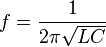$f = \frac{1}{2 \pi \sqrt{LC}}$

This is the frequency at which resonance occurs in an LC circuit.

### Other applications

#### Sensing

Most capacitors are designed to maintain a fixed physical structure. However, various things can change the structure of the capacitor—the resulting change in capacitance can be used to sense those things.

Changing the dielectric: The effects of varying the physical and/or electrical characteristics of the dielectric can also be of use. Capacitors with an exposed and porous dielectric can be used to measure humidity in air.

Changing the distance between the plates: Capacitors are used to accurately measure the fuel level in airplanes. Capacitors with a flexible plate can be used to measure strain or pressure. Capacitors are used as the sensor in condenser microphones, where one plate is moved by air pressure, relative to the fixed position of the other plate. Some accelerometers use MEMS capacitors etched on a chip to measure the magnitude and direction of the acceleration vector. They are used to detect changes in acceleration, e.g., as tilt sensors or to detect free fall, as sensors triggering airbag deployment, and in many other applications. They are also used in fingerprint sensors.

#### Pulsed power and weapons

Groups of large, specially constructed, low-inductance high-voltage capacitors (capacitor banks) are used to supply huge pulses of current for many pulsed power applications. These include electromagnetic forming, Marx generator , pulsed lasers (especially TEA lasers), pulse forming networks, radar, fusion research, and particle accelerators.

Large capacitor banks are used as energy sources for the exploding-bridgewire detonators or slapper detonators in nuclear weapons and other specialty weapons. Experimental work is under way using banks of capacitors as power sources for electromagnetic armour and electromagnetic railguns or coilguns.

## Hazards and safety

Capacitors may retain a charge long after power is removed from a circuit; this charge can cause shocks (sometimes fatal) or damage to connected equipment. For example, even a seemingly innocuous device such as a disposable camera flash unit powered by a 1.5 volt AA battery contains a capacitor which may be charged to over 300 volts. This is easily capable of delivering an extremely painful, and possibly lethal shock.

Care must be taken to ensure that any large or high-voltage capacitor is properly discharged before servicing the containing equipment. For safety purposes, all large capacitors should be discharged before handling. For board-level capacitors, this is done by placing a bleeder resistor across the terminals, whose resistance is large enough that the leakage current will not affect the circuit, but small enough to discharge the capacitor shortly after power is removed. High-voltage capacitors should be stored with the terminals shorted, since temporarily discharged capacitors can develop potentially dangerous voltages when the terminals are left open-circuited.

Large oil-filled old capacitors must be disposed of properly as some contain polychlorinated biphenyls (PCBs). It is known that waste PCBs can leak into groundwater under landfills. If consumed by drinking contaminated water, PCBs are carcinogenic, even in very tiny amounts. If the capacitor is physically large it is more likely to be dangerous and may require precautions in addition to those described above. New electrical components are no longer produced with PCBs. ("PCB" in electronics usually means printed circuit board, but the above usage is an exception.) Capacitors containing PCB were labelled as containing "Askarel" and several other trade names.

### High-voltage

Above and beyond usual hazards associated with working with high voltage, high energy circuits, there are a number of dangers that are specific to high voltage capacitors. High voltage capacitors may catastrophically fail when subjected to voltages or currents beyond their rating, or as they reach their normal end of life. Dielectric or metal interconnection failures may create arcing within oil-filled units that vaporizes dielectric fluid, resulting in case bulging, rupture, or even an explosion that disperses flammable oil, starts fires, and damages nearby equipment. Rigid cased cylindrical glass or plastic cases are more prone to explosive rupture than rectangular cases due to an inability to easily expand under pressure. Capacitors used in RF or sustained high current applications can overheat, especially in the center of the capacitor rolls. The trapped heat may cause rapid interior heating and destruction, even though the outer case remains relatively cool. Capacitors used within high energy capacitor banks can violently explode when a fault in one capacitor causes sudden dumping of energy stored in the rest of the bank into the failing unit. And, high voltage vacuum capacitors can generate soft X-rays even during normal operation. Proper containment, fusing, and preventative maintenance can help to minimize these hazards.

High voltage capacitors can benefit from a pre-charge to limit in-rush currents at power-up of HVDC circuits. This will extend the life of the component and may mitigate high voltage hazards.

## Physics

A capacitor consists of two conductive electrodes, or plates, separated by an insulator.

### CapacitanceWhen electric charge accumulates on the plates, an electric field is created in the region between the plates that is proportional to the amount of accumulated charge. This electric field creates a potential difference V = E·d between the plates of this simple parallel-plate capacitor.

The capacitor's capacitance (C) is a measure of the amount of charge (Q) stored on each plate for a given potential difference or voltage (V) which appears between the plates: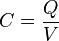$C = {Q \over V}$

In SI units, a capacitor has a capacitance of one farad when one coulomb of charge causes a potential difference of one volt across the plates. Since the farad is a very large unit, values of capacitors are usually expressed in microfarads (µF), nanofarads (nF), or picofarads (pF).

The capacitance is proportional to the surface area of the conducting plate and inversely proportional to the distance between the plates. It is also proportional to the permittivity of the dielectric (that is, non-conducting) substance that separates the plates.

The capacitance of a parallel-plate capacitor is given by: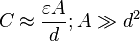$C \approx \frac{\varepsilon A}{d}; A \gg d^2$ 

where ε is the permittivity of the dielectric, A is the area of the plates and d is the spacing between them.

In the diagram, the rotated molecules create an opposing electric field that partially cancels the field created by the plates, a process called dielectric polarization.

### Stored energy

As opposite charges accumulate on the plates of a capacitor due to the separation of charge, a voltage develops across the capacitor owing to the electric field of these charges. Ever-increasing work must be done against this ever-increasing electric field as more charge is separated. The energy (measured in joules, in SI) stored in a capacitor is equal to the amount of work required to establish the voltage across the capacitor, and therefore the electric field. The maximum energy that can be safely stored in a particular capacitor is limited by the maximum electric field that the dielectric can withstand before it breaks down. Therefore, all capacitors made with the same dielectric have about the same maximum energy density (joules of energy per cubic meter).

### Hydraulic model

As electrical circuitry can be modeled by fluid flow, a capacitor can be modeled as a chamber with a flexible diaphragm separating the input from the output. As can be determined intuitively as well as mathematically, this provides the correct characteristics

• The pressure difference (voltage difference) across the unit is proportional to the integral of the current
• A steady state current cannot pass through it because applying too much pressure, above the maximum pressure, will destroy it.
• But a transient pulse or alternating current can be transmitted
• The capacitance of units connected in parallel is equivalent to the sum of their individual capacitances

## Electrical circuitsThe electrons within dielectric molecules are influenced by the electric field, causing the molecules to rotate slightly from their equilibrium positions. The air gap is shown for clarity; in a real capacitor, the dielectric is in direct contact with the plates. Capacitors also allow AC current to flow and blocks DC current.

### DC sources

Electrons cannot easily pass directly across the dielectric from one plate of the capacitor to the other as the dielectric is carefully chosen so that it is a good insulator. When there is a current through a capacitor, electrons accumulate on one plate and electrons are removed from the other plate. This process is commonly called 'charging' the capacitor—even though the capacitor is at all times electrically neutral. In fact, the current through the capacitor results in the separation of electric charge, rather than the accumulation of electric charge. This separation of charge causes an electric field to develop between the plates of the capacitor giving rise to voltage across the plates. This voltage V is directly proportional to the amount of charge separated Q. Since the current I through the capacitor is the rate at which charge Q is forced through the capacitor (dQ/dt), this can be expressed mathematically as: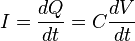$I = \frac{dQ}{dt} = C\frac{dV}{dt}$ where I is the current flowing in the conventional direction, measured in amperes, dV/dt is the time derivative of voltage, measured in volts per second, and C is the capacitance in farads.

For circuits with a constant (DC) voltage source, the voltage across the capacitor cannot exceed the voltage of the source. (Unless the circuit includes a switch and an inductor, as in SMPS, or a switch and some diodes, as in a charge pump). Thus, an equilibrium is reached where the voltage across the capacitor is constant and the current through the capacitor is zero. For this reason, it is commonly said that capacitors block DC.

Capacitors also have a good use in hobby robot circuits powered by DC.

### AC sources

The current through a capacitor due to an AC source reverses direction periodically. That is, the alternating current alternately charges the plates: first in one direction and then the other. With the exception of the instant that the current changes direction, the capacitor current is non-zero at all times during a cycle. For this reason, it is commonly said that capacitors "pass" AC. However, at no time do electrons actually cross between the plates, unless the dielectric breaks down. Such a situation would involve physical damage to the capacitor and likely to the circuit involved as well.

Since the voltage across a capacitor is proportional to the integral of the current, as shown above, with sine waves in AC or signal circuits this results in a phase difference of 90 degrees, the current leading the voltage phase angle. It can be shown that the AC voltage across the capacitor is in quadrature with the alternating current through the capacitor. That is, the voltage and current are 'out-of-phase' by a quarter cycle. The amplitude of the voltage depends on the amplitude of the current divided by the product of the frequency of the current with the capacitance, C.

#### Impedance

Impedance is analogous to the resistance of a resistor. The impedance of a capacitor is inversely proportional to the frequency—that is, for very high-frequency alternating currents the reactance approaches zero—so that a capacitor is nearly a short circuit to a very high frequency AC source. Conversely, for very low frequency alternating currents, the reactance increases without bound so that a capacitor is nearly an open circuit to a very low frequency AC source. This frequency dependent behavior accounts for most uses of the capacitor.

Reactance is so called because the capacitor doesn't dissipate power, but merely stores energy. In electrical circuits, as in mechanics, there are two types of load, resistive and reactive. Resistive loads (analogous to an object sliding on a rough surface) dissipate the energy delivered by the circuit, ultimately by electromagnetic emission, while reactive loads (analogous to a spring or frictionless moving object) store this energy, ultimately delivering the energy back to the circuit.

Also significant is that the impedance is inversely proportional to the capacitance, unlike resistors and inductors for which impedances are linearly proportional to resistance and inductance respectively. This is why the series and shunt impedance formulae (given below) are the inverse of the resistive case. In series, impedances sum. In parallel, conductances sum.

#### Laplace equivalent (s-domain)

When using the Laplace transform in circuit analysis, the capacitive impedance is represented in the s domain by: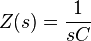$Z(s)=\frac{1}{sC}$

where C is the capacitance, and s (= σ+jω) is the complex frequency.

### Displacement current

The physicist James Clerk Maxwell invented the concept of displacement current, dD/dt, to make Ampere's law consistent with conservation of charge in cases where charge is accumulating as in a capacitor. He interpreted this as a real motion of charges, even in vacuum, where he supposed that it corresponded to motion of dipole charges in the ether. Although this interpretation has been abandoned, Maxwell's correction to Ampere's law remains valid.

### Networks

#### Series or parallel arrangements

Capacitors in a parallel configuration each have the same potential difference (voltage). Their total capacitance (Ceq) is given by: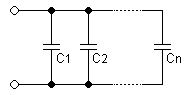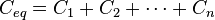$C_{eq} = C_1 + C_2 + \cdots + C_n \,$

The reason for putting capacitors in parallel is to increase the total amount of charge stored. In other words, increasing the capacitance also increases the amount of energy that can be stored. Its expression is: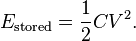$E_\mathrm{stored} = {1 \over 2} C V^2 .$

The current through capacitors in series stays the same, but the voltage across each capacitor can be different. The sum of the potential differences (voltage) is equal to the total voltage. Their total capacitance is given by:$\frac{1}{C_{eq}} = \frac{1}{C_1} + \frac{1}{C_2} + \cdots + \frac{1}{C_n}$

In parallel the effective area of the combined capacitor has increased, increasing the overall capacitance. While in series, the distance between the plates has effectively been increased, reducing the overall capacitance.

In practice capacitors will be placed in series as a means of economically obtaining very high voltage capacitors, for example for smoothing ripples in a high voltage power supply. Three "600 volt maximum" capacitors in series, will increase their overall working voltage to 1800 volts. This is of course offset by the capacitance obtained being only one third of the value of the capacitors used. This can be countered by connecting 3 of these series set-ups in parallel, resulting in a 3x3 matrix of capacitors with the same overall capacitance as an individual capacitor but operable under three times the voltage. In this application, a large resistor would be connected across each capacitor to ensure that the total voltage is divided equally across each capacitor and also to discharge the capacitors for safety when the equipment is not in use.

Another application is for use of polarized capacitors in alternating current circuits; the capacitors are connected in series, in reverse polarity, so that at any given time one of the capacitors is not conducting...

### Capacitor/inductor duality

In mathematical terms, the ideal capacitor can be considered as an inverse of the ideal inductor, because the voltage-current equations of the two devices can be transformed into one another by exchanging the voltage and current terms. Just as two or more inductors can be magnetically coupled to make a transformer, two or more charged conductors can be electrostatically coupled to make a capacitor. The mutual capacitance of two conductors is defined as the current that flows in one when the voltage across the other changes by unit voltage in unit time.